Get instant live expert help with Excel or Google Sheets“My Excelchat expert helped me in less than 20 minutes, saving me what would have been 5 hours of work!”

#### Post your problem and you’ll get expert help in seconds.

Your message must be at least 40 characters
Our professional experts are available now. Your privacy is guaranteed.

# How to Create A Control Chart – Excelchat

We can use the statistical process control chart in Excel to study how processes or data changes occur over time. The Control chart has four lines including; a straight-line representing average, the data and a lower control limit (LCL) and an upper control limit (UCL). In this tutorial, we will learn how to create a control chart for analyzing data.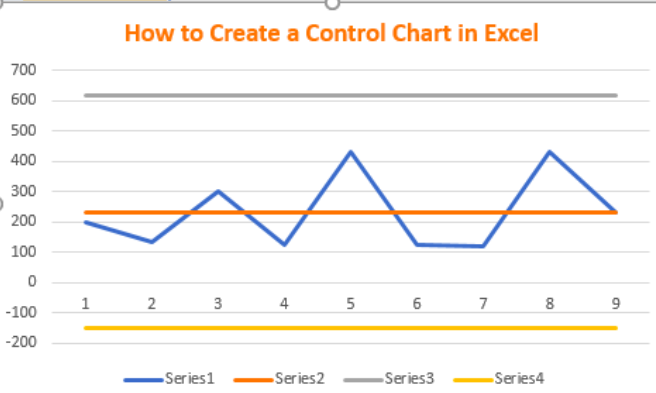Figure 1 – How to make a statistical process control chart in excel

## Creating Control Charts

• We will begin by setting up our data as shown in Figure 2
• Column A will contain the Date (will not be used in creating control chart)
• Column B contains our Sample Data
• Column C will contain the Control Line ( Mean or Average)
• Column D will contain the UCL (Upper Control Limit)
• Column E will contain the LCL (Lower Control Limit)

•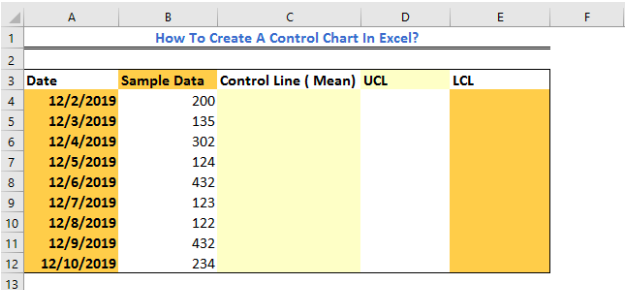Figure 2 – Data to create a control chart

• Below our Table, we will set up a mini table. In Cells A14 and Cell A15, we will enter Mean and Std. Dev (standard deviation) respectively.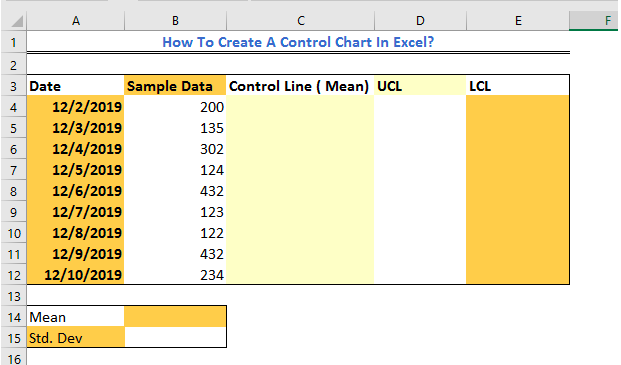Figure 3 – How to create a control chart

• We will click on Cell B14. We will go to the Formula Tab and select the small Arrow beside the Autosum to view the drop-down menu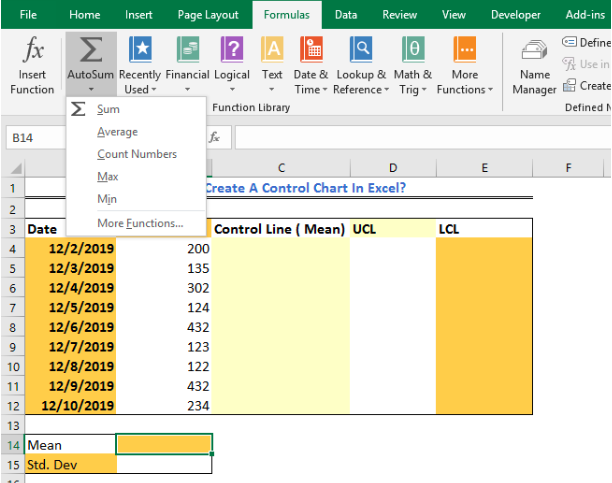Figure 4 – How to create a control chart

• In the drop-down menu, we will select Average and highlight Cells B4:B12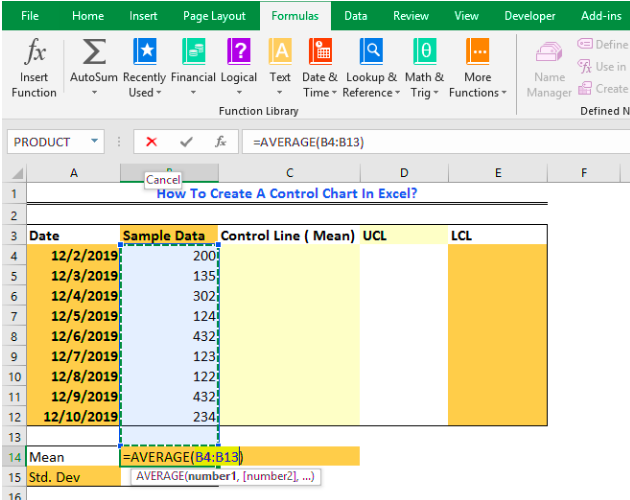Figure 5 – How to create a control chart

• We will press Enter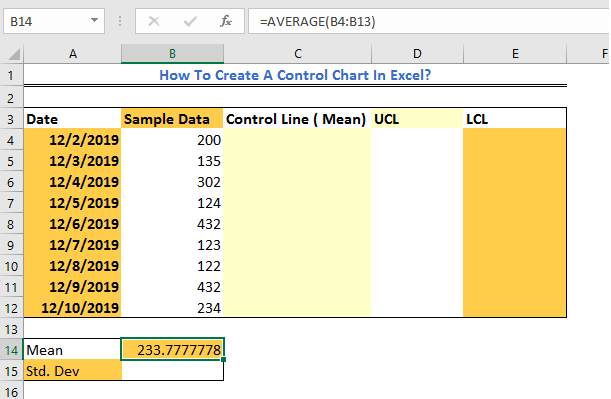Figure 6 – How to make a control chart

• We will click again, this time in Cell B15, go the Formula Tab again, and click the small Arrow next to the Autosum. In the drop-down menu, we will select More Functions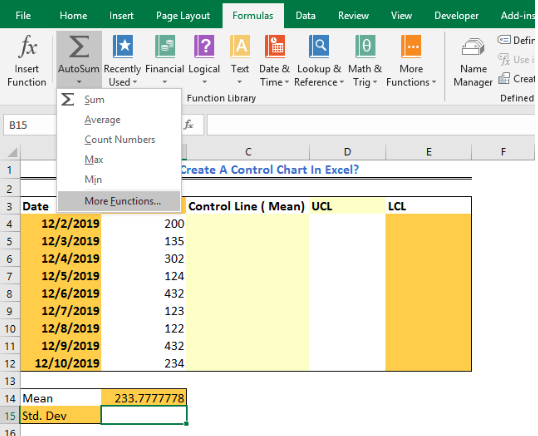Figure 7 – How to control charts in excel

• In the Insert Function dialog Window, we will select STDEV and click OK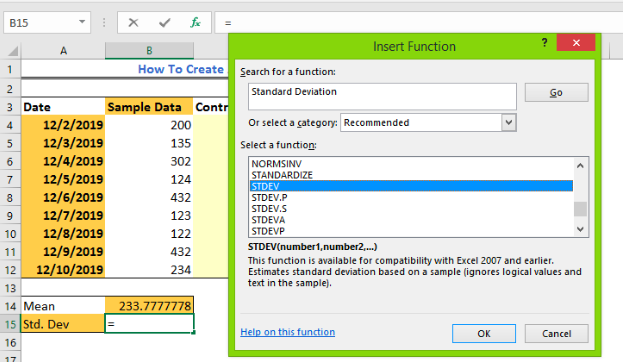Figure 8 – Creating control charts

• Again, we will highlight the Cells B4:B12 containing the data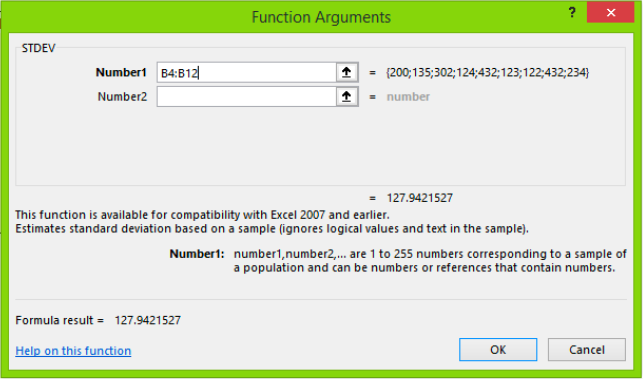Figure 9 – Making control charts

• We will press Enter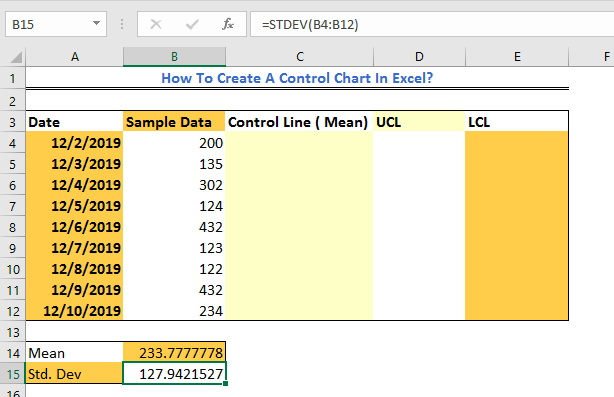Figure 10 – How to create control chart

• In Cell C4, we will enter the cell reference for the Cell contain the average, i.e. we will enter =B\$14.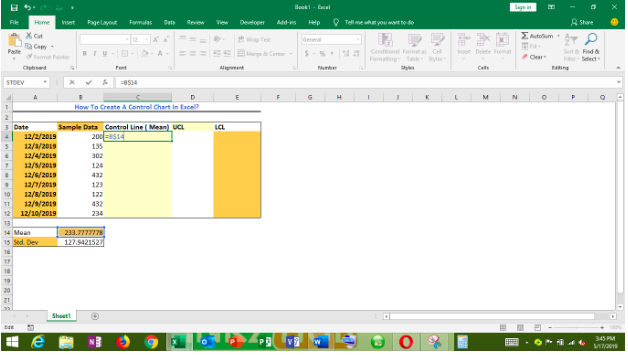Figure 11 – Making control charts in Excel

• We will press Enter. We will double-click the fill handle tool (the small box at the bottom-right as shown in Cell C5 of the figure below) and drag down the column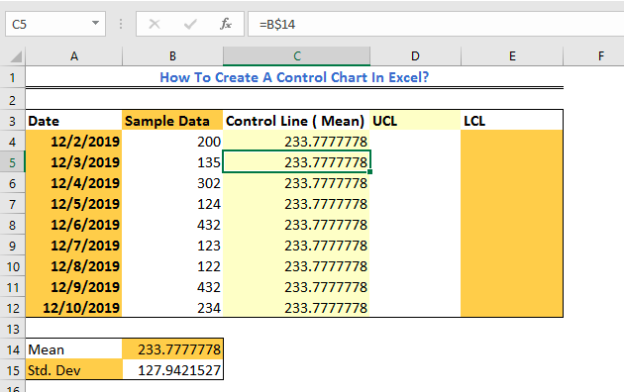Figure 12 – How to create a control chart

• In Cell D4, we will add Average to STDEV multiplied by 3, which means we will have the formula below:

`=B\$14+(B\$15*3)`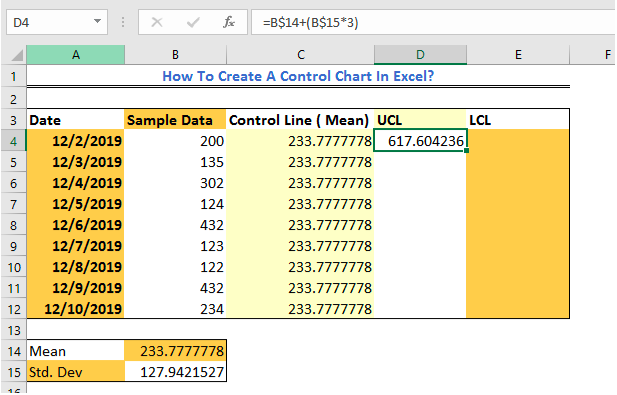Figure 13 – Making a control chart

• We will use the Fill handle tool to fill the result down the column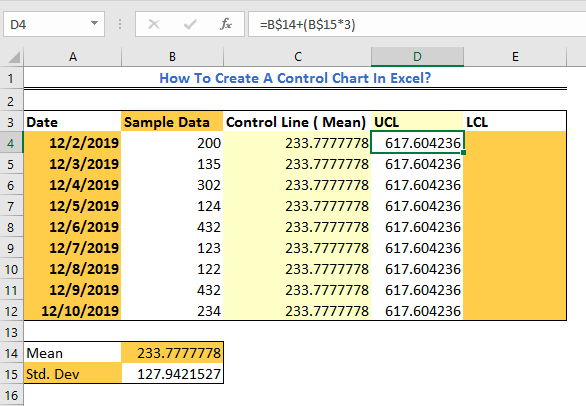Figure 14 – Control charts in Excel

• In Cell E4, we will enter the formula below:

`=B\$14-(B\$15*3)`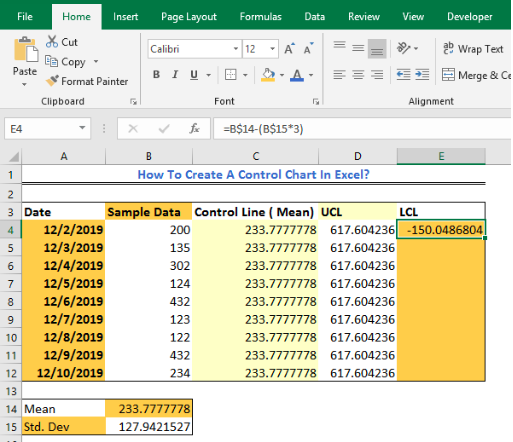Figure 15 – Statistical process control

• We will use the fill handle tool to enter the result down Column E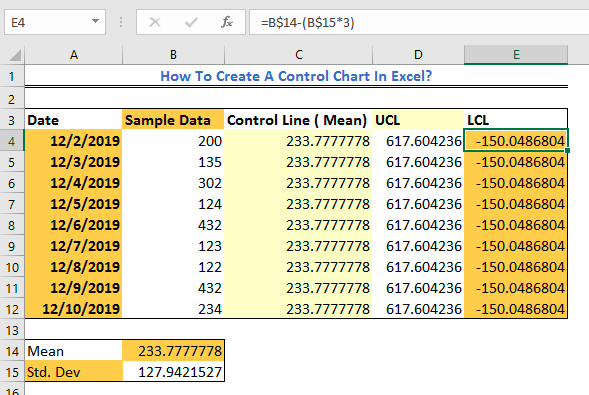Figure 16 – Process control chart in excel

• We will highlight range B4:E12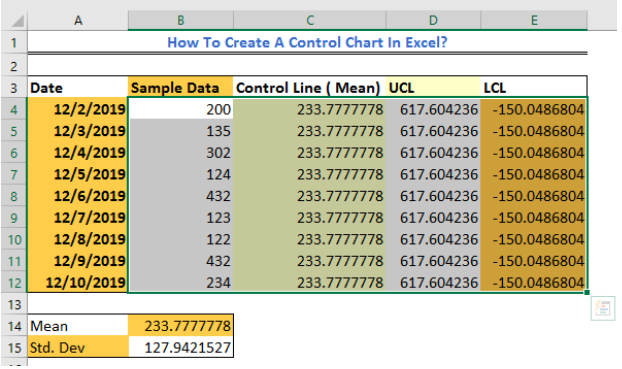Figure 17 – Create a control chart in Excel

• Next, we will go to the Insert Tab and select the Line chart from the Chart Group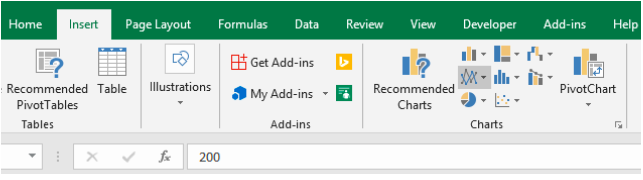Figure 18 – Control chart in Excel

• In the drop-down menu, we will select the first Line Chart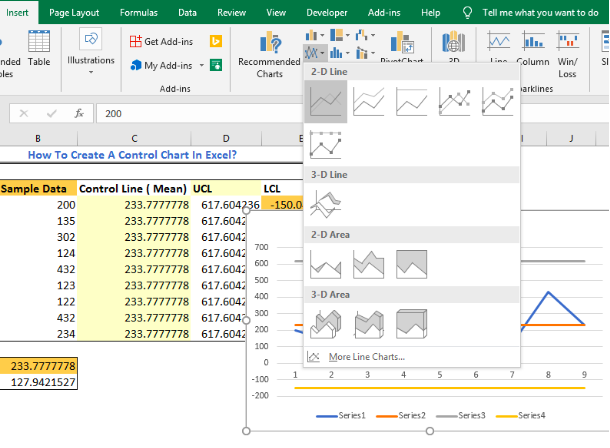Figure 19 – Control chart in Excel

• We can now add a chart title, change or modify our Control chart as desired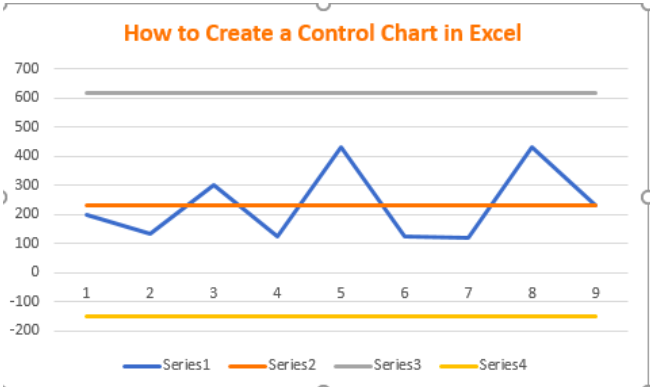Figure 20 – Excel Control Chart

## Instant Connection to an Excel Expert

Most of the time, the problem you will need to solve will be more complex than a simple application of a formula or function. If you want to save hours of research and frustration, try our live Excelchat service! Our Excel Experts are available 24/7 to answer any Excel question you may have. We guarantee a connection within 30 seconds and a customized solution within 20 minutes.